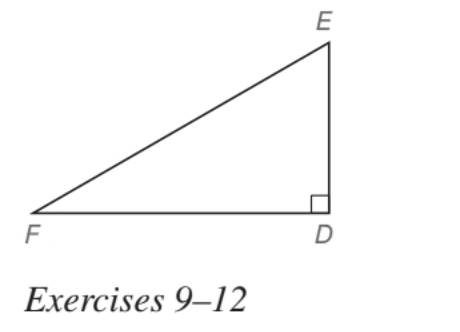Chapter 5.5, Problem 12EElementary Geometry For College St...

7th Edition
Alexander + 2 others
ISBN: 9781337614085

Solutions

Chapter
SectionElementary Geometry For College St...

7th Edition
Alexander + 2 others
ISBN: 9781337614085
Textbook Problem

In Exercises 5 to 22, find the missing lengths. Give your answers in both simplest radical form and as approximations correct two decimal places. Given: Right ∆ D E F with m ∠ E = 2 ċ m ∠ F and E F = 12 3 Find: D E and D FTo determine

To find:

DF and DF of the right DEF with mE=2·mF and EF=123.

Explanation

Approach:

For a right triangle, for which the measure of the interior angles 30°, 60°, and 90°; if ‘a’ is the length of measure of the shorter leg; opposite to the angle 30°, then the length of the other two sides is given by

Length of the longer leg (opposite to 60°) =a3

Length of the hypotenuse (opposite to 90°)=2a.

In general

Length of the longer leg =3× (Length of the shorter leg)

Length of the hypotenuse =2× (Length of the shorter leg)

Calculation:

Given,

A right triangle DEF

mE=2·mF

EF=123

Since;mE=2mF, we have by the property of the triangle that the sum of all the three interior angles is 180°,

mD+mE+mF=180°

mD+2mE+mF=180°

Since, the triangle DEF is having its right angle at D, mD=90°

Thus,

90°+2mF+mF=180°

90°+3mF=180°

Add; -90° on both sides,

90°+3mF+-90°=180°+-90°

90°+-90°+3mF=180°+-90°

3mF=90°

Divide; by 3 on both side,

3mF3=90°3

mF=30°

Thus,

mE=2 mF

=2(30°)

mE=60°

Thus, the triangle DEF become

Still sussing out bartleby?

Check out a sample textbook solution.

See a sample solution

The Solution to Your Study Problems

Bartleby provides explanations to thousands of textbook problems written by our experts, many with advanced degrees!

Get Started

Convert the expressions in Exercises 6584 to power form. 4x23+x3/2623x2

Finite Mathematics and Applied Calculus (MindTap Course List)

Prove that |ab| = |a||b|. [Hint: Use Equation 4.]

Single Variable Calculus: Early Transcendentals

True or False: If f(x) = F(x), then baf(x)dx=F(b)F(a).

Study Guide for Stewart's Single Variable Calculus: Early Transcendentals, 8th

For , f(x) = 0 2 3 f(3) does not exist

Study Guide for Stewart's Multivariable Calculus, 8th

In Exercises 57-62, Use Venn diagram to illustrate each statement. (AB)c=AcBc

Finite Mathematics for the Managerial, Life, and Social Sciences